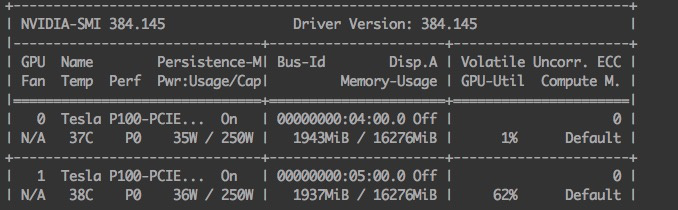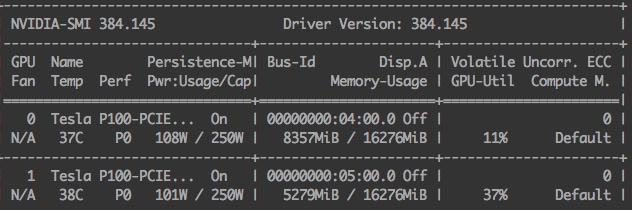# Gpu memory increase ,second forward use far more gpu memory

when run code :

``````import torch
from torch import nn

class Test(nn.Module):
def __init__(self, in_ch , n_classes):
super(ResASPSPNetEncoder, self).__init__()
self.conv1 = nn.Conv2d(1280,1024,1)
def forward(self, x):
x = self.conv1(x)
return x

model = Test(in_ch=7,n_classes =3)
model = torch.nn.DataParallel(model,device_ids=list(range(2))).cuda()
x = torch.zeros((8,1280,256,256),dtype=torch.float)

for i in range(2):
y = model(x)
print(i)
# set breakpoint，use nvidia-smi to watch GPU memory
``````

when set breakpoint after line “y = model(x)”, and use nvidia-smi to watch GPU memory,
second use far more gpu memory , why ？
first forward :second forward :THX

Because your model is set to `train` mode by default, pytorch is probably keeping the forward flow of the network with the specific inputs for future gradient computations. If you only want to use your model for inference, you should try calling `model.eval()` first, and also use `with torch.no_grad():` before all calls that should not compute gradients.

If you want to train, however, you should try with an optimizer and loss function and follow the usual procedure, i.e:

``````model.train()
for i in range(2):
# forward
y = model(x)
# reset previous gradients (free memory)